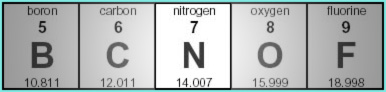Knowledge database: Stoichiometry: Relative atomic and molecular mass Relative atomic mass is a number that shows us how many times is the mass of a given atom greater than 1/12 of the mass of a carbon atom (with the mass number of 12), which is also known as the unified atomic mass unit. This unit is signified with u or m(u) and equals 1.6605x10-27 kg. Thus, the relative atomic mass of an element can be calculated by taking the mass of the atom and dividing it by the unified atomic mass unit. Likewise, if one knows the relative atomic mass of an atom, one can calculate its mass by multiplying the relative atomic mass by the unified atomic mass unit.On the picture above, a part of the standard periodic table of elements is shown. In the case of nitrogen, the relative atomic mass is 14.007. This value is usually placed beneath the symbol of the element. Relative molecular mass is analogous to the relative atomic mass. The only difference here is that one doesn't deal with atoms as particles, but with molecules.